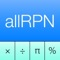allRPNCalc Calculator
See on store
Category
Store
Last Updated
2019, Feb 01
Publisher
Visibility Score
0

Jul, 2020
Worldwide

Jul, 2020
Worldwide

## Description

allRPNCalc is a versatile calculator that uses the powerful Reverse Polish Notation logic system combined with an automated 4 level stack to compute answers to complex mathematical problems. The keyboard layout and display have been optimized for use on an iPhone,iPod Touch and iPad. Large keys with sound feed back give you typing confidence and the last function keyed is always displayed. Functions are logically laid out to eliminate multiple key entry for most problems. Display: Results are displayed in fixed, fraction, scientific or engineering notation with from 0 to 14 significant digits displayed. In programmer’s mode hex, decimal, octal or binary (with windowing) can be displayed. Calculations are carried out in industry standard IEEE double format with 14+ significant digits and exponents ranging +/-308. Math: +, -, ×, ÷, ln, log, e^x, 10^x, y^x, x², x³, 1/x, √x, ∛x, fraction part, integer part, absolute value, random, seed, mod Trigonometry: sin, cos, tan, asin, acos, atan, sinh, cosh, tanh, asinh, acosh, atanh, to radians, to degrees, to rectangular, to polar, to hours, to hours minutes seconds, hours minutes seconds add and subtract, triangle solver, π, π/2. Trig functions can be computed in radians, degrees or gradians. Statistics: sum plus, sum minus, mean, weighted mean, standard deviation, population standard deviation, linear estimate x, y and correlation coefficient, combination, permutation, factorial (gamma), percent, delta percent, percent of total Programer’s functions: and, or, xor, not, shift left, shift right, rotate left, rotate right, word sizes 8, 16, 32, 48 Conversions: 47 world currencies and over 100 commonly used metric, english and other conversion factors used in scientific, engineering and financial calculations. Financial: time value of money calculations, n, i, pv, pmt, fv, depreciation (straight line, sum-of-years, declining balance), bond price and yield to maturity, amortization, net present value, internal rate of return, up to 80 cash flows, days between dates, date plus days, rounding. Storage: 4 level automatic stack with lastX, exchange and roll down, 20 general purpose storage registers, register arithmetic +,-,×,÷ , 6 dedicated registers for statistics, 80 cash flow entries, 5 financial registers.

## Reviews

#### Try MobileAction free for a week!

No credit card required.